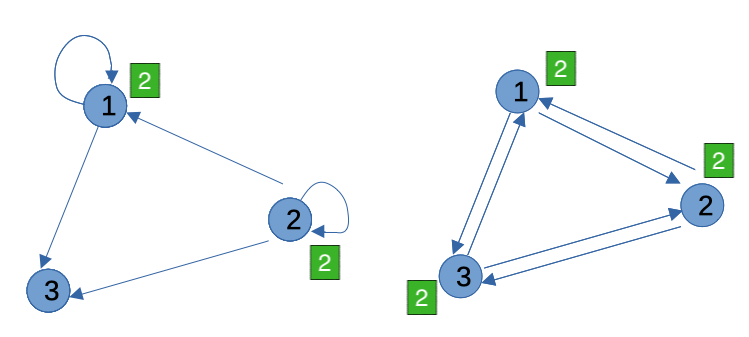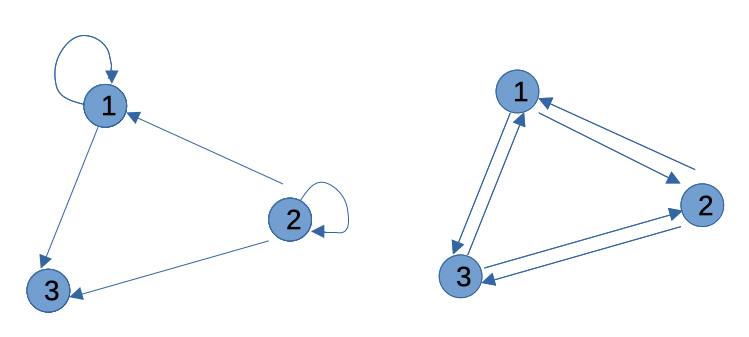×

Search anything:

# Time Complexity of Topological Sort

#### Time Complexity Graph Algorithms topological sortGet this book -> Problems on Array: For Interviews and Competitive Programming

In this article at OpenGenus, you will learn about Time Complexity of Topological Sort. Specifically, the version of Topological Sort using Khan's Algorithm, which uses Breath First Search with a Queue. The tutorial will cover the mathematical runtime analysis of the best, average and worst cases.

In short:

Best case time complexity: Θ(E+V)
Average case time complexity: Θ(E+V)
Worst case time complexity: Θ(E+V)

1. What is Topological Sort used for?
2. Overview of Topological Sort
3. Topological Sort using Khan's Algorithm in Python
4. Time Complexity Analysis
5. Best, Average, Worst Case Time Complexity Analysis

# 1. What is Topological Sort used for?

##### Topological Sort Usage

Topological Sort is used in many real world applications. For example:

• Course scheduling, ex. determining prerequisites taken in a certina order
• Make files, ex. determining the order of compilation tasks
• Instruction dependency in compiler
• Deadlock detection in operating system
• Data serialization
##### Basics to know:

Topological Sort:

• A topological sort takes a Directed Acyclic Graph (DAG) and sorts the nodes in linear order.
• A DAG can have different topological ordering depending on which child node is selected.

Cycle Graph (Circular Graph)

• Graphs with cycles can't be linearly ordered, thus can't be sorted by topological sort.

Directed Acyclic Graph (DAG)

• It's a directed graph with no directed cycles.

# 2. Overview of Topological Sort

##### Basic Idea In One Sentence

Identify and enqueue nodes with 0 incoming edges, dequeue them one-by-one, update their neighbors' incoming edges (indegree), and append the removed nodes to a sorted list. Repeat.

##### In Plain English
1. Identify number of incoming edges (indegrees) for each node.
2. Add nodes with indegree=0 to a queue.
3. This part is the core of the topo sort. As long as there are nodes in the queue, keep looping.
3-1. Dequeue a node from the queue, and add it to the topo ordered list.
3-2. Decrement the indegree of this node's neighbors. If a neighbor's indegree becomes 0, append it to the queue.
4. The loop ends after we run out of nodes with indegree=0.
5. Finally, we check if the input graph is able to be sorted by checking if the length of the sorted list is the same as the number of input nodes.
5-1. If yes, return the sorted list.
5-2. If no, there is a cycle in the input graph.

# 3. Topological Sort using Khan's Algorithm in Python

``````from collections import defaultdict, deque

class Graph(object):
def topoSortBfs(self, numNodes, edgeList):

# Create an adjacency list for each node
adjList = defaultdict(list)  # dict of list
# Create indegree for each node to track incoming edges
indegree = defaultdict(int) # dict of int
for src, dst in edgeList:
indegree[dst] += 1
print("indegree:", indegree)

# Enqueue nodes that have 0 incoming edges (indegree=0) to start
# Khan's Algo
queue = deque()
for node in range(numNodes):
if node not in indegree:
queue.append(node)
print("queue:", queue)

# Keep looping until the queue is empty.
# Whenever we dequeue a node, we append it to the topo ordered list.
topoOrder = []
while queue:
src = queue.popleft()

# Deduct indegree by 1 for all neighbors of this node.
# If a neighbor has 0 incoming edge, append it to queue.
indegree[neighbor] -= 1
if indegree[neighbor] == 0:
queue.append(neighbor)

topoOrder.append(src)

# Check if all nodes are handled, or there're nodes left meaning
# there is a cycle.

g = Graph()
numNodes = 6

# This graph is a DAG
edgeList_1 = [[0,2], [0,3], [3,1], [4,1], [4,2], [5,0], [5,2]]
res = g.topoSortBfs(numNodes, edgeList_1)
print("Topo Order: ", res)

print("- - - - - - - - - - - - - - - - -\n")
# This graph has a cycle
edgeList_2 = [[0,2], [0,3], [3,1], [4,1], [4,2], [5,0], [5,2], [1,4]]
res = g.topoSortBfs(numNodes, edgeList_2)
print("Topo Order: ", res)
``````

# 4. Time Complexity Analysis

##### Time Analysis

Note: E: Edge, V: Node

(1) We look at each directed edge to
(a) build an adjacency list: O(E)
(b) determine indegree of each node: O(E)
=> O(E)

(2) Enqueue nodes with 0 incoming edges (indegree=0) by going through all the nodes
=> O(V)

(3) Now we move on to the while loop that's the core of the Topo Sort. It is supposed to go through at Max(N - 1) nodes in the input graph if the graph is a DAG (Directed Acyclic Graph). It's Max(N - 1) nodes because at least 1 node with indegree=0 is enqueued to kickstart the loop.
=> O(V)

Note:
If there are no nodes with indegree=0, it means there are cycles in the graph, and the graph can't be sorted. And we know that if the graph has cycles, the loop doesn't go through all the nodes. But since we are talking about graphes that can be sorted, all nodes will be enqueued.(4) Inside the while loop, for each node dequeued, deduct the indegree of its neighbors by 1. All edges will be decremented once eventually. So the number of times the decrement operation is performed is equal to the number of edges.: O(E)

Note:
This is where some people get it wrong. They might think a node could connect to up to N-1 nodes excluding itself, so the graph doesn't create a cycle, but it's wrong. See this example for clarification:(5) Check if the data is sorted: O(1)
Compare number of nodes in the topo order list to input numNodes.

=> Total Time Complexity: O(E + V)

# 5. Best, Average, Worst Case Time Complexity Analysis

Best case time complexity: Θ(E+V)
Average case time complexity: Θ(E+V)
Worst case time complexity: Θ(E+V)

Since we perform the same steps regardless of how the elements are organized, the best, worst and average cases time complexity of this algorithm are the same.